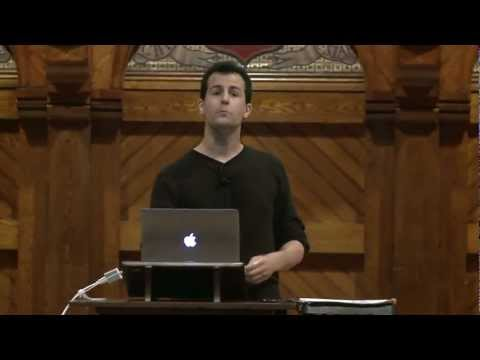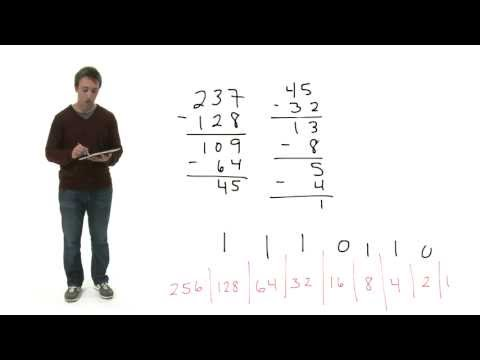# Binary

## Notes

Everything that goes on under the hood of a computer is done in binary -- the language of 0s and 1s.

If we have only two numbers, it's very easy to represent them in the physical world using electricity. You can think of each binary digit as a switch or a light bulb that can be either on or off, where by convention 0 is thought of as "off" and 1 is "on".

Let's think more carefully about decimal notation.

To represent the number 163, we've got a 3 in the 1s place (100), a 6 in the 10s place (101), and a 1 in the 100s place (102). You get 163 when you multiply these digits by their respective powers of 10 and sum them.

To represent this same number in binary, you'll need a 1 in the 1s place (20), a 1 in the 2s place (21), a 1 in the 32s place (25), and a 1 in the 128s place (27).

You get 163 when you multiply these digits by their respective powers of 2 and sum them.

Let's count to 5 in binary! Note how we can multiply the binary digits by their respective powers of two and sum them to convert to a decimal value.

Addition and subtraction in binary works the same way as in decimal. Start on the right, and carry the 1s as needed.## Slides ( / )## Decimal to Binary

Write a program that takes a positive decimal value as input and prints out that number in binary notation.

``````jharvard@run.cs50.net (~): ./a.out
Please enter a positive decimal value: 45
101101``````

Try out some pseudocode here!
```#include <cs50.h>
#include <stdio.h>

int main (int argc, string argv[])
{

}
```

Try these problems for practice:
1. Convert 10101101 to decimal notation.

2. Convert 365 to binary notation.

3. Perform the following calculation in binary. Be sure to show your work (i.e., any 1s carried).

## Videos#### Wednesday, Week 0

An introduction to binary#### Nate's Binary Short

Nate gives a general overview